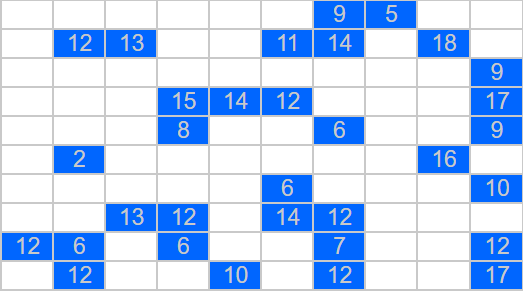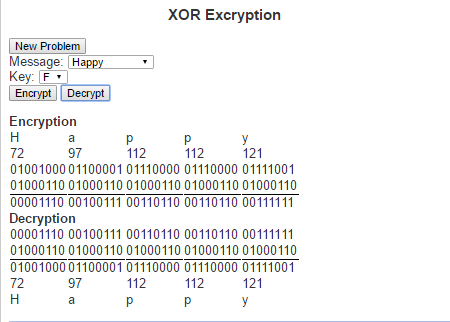# Range Puzzles

Range PuzzlesI have always liked puzzles. I really enjoy discovering a new puzzle. Sometimes when I have discovered a new puzzle, I enjoy it so much that I can’t seem to do enough of them. In such cases, I quickly run out of these puzzles to do and find myself looking for either a new puzzle or a way to generate them on my own.

Such was the case when I discovered the “Range Puzzles”. The rules are simple:

Every cell is marked either blue or gray
No two gray cells can be next to one another
The grid must be a connected (i.e. there is always a path from every cell to every other cell using horizontal and vertical connections.
Some cells have a number inside. This indicates the number of cells that can be viewed (horizontally and vertically in both directions) by this cell, including the cell itself.

To try out these puzzles, visit Range Puzzles at LEARNINGlover.com

# Lets Learn About XOR EncryptionOne of the more common things about this generation is the constant desire to write up (type) their thoughts. So many of the conversations from my high school days were long lasting, but quickly forgotten. Today’s generation is much more likely to blog, tweet, write status updates or simply open up a notepad file and write up their thoughts after such a conversation.

When we feel that our thoughts are not ready for public eyes (maybe you want to run your idea by the Patent and Trademark Office before speaking about it) we may seek some form of security to ensure that they stay private. An old fashioned way of doing this was to write in a diary and enclosed it within a lock and key. The mathematical field of encryption also tries to grant privacy by encoding messages so that only people with the necessary information can read them.

The type of encryption I want to speak about today is called XOR encryption. It is based on the logical operation called “exclusive or” (hence the name XOR). The exclusive or operation is true between two logical statements if exactly one of the two statements is true, but not both statement. This can be represented with the following truth table

 Input 1 Input2 XOR Result T T F T F T F T T F F F

XOR Encryption is particularly useful in this day and age because we every character we type is understood by the computer as a sequence of zeros and ones. The current standard encoding that is used is Unicode (also known as UTF-8). Under this encoding the letter ‘a’ is represented as the binary string ‘01100001’. Similarly every letter, number and special character can be represented as its own binary string. These binary strings are just an assignment of numbers to these characters so that we can to help represent them in the computer. The numbers can the be thought of in base 10, which is how we generally think about numbers, or in base 2 which is how computers generally work with numbers (or a number of other ways). The way we would use these binary strings in encoding is first by translating a text from human-readable text to machine readable text via its binary string. For example, the word “Invincible”, we would get the following binary strings:

 Letter Unicode in base 10 Unicode in base 2 I 73 01001001 n 110 01101110 v 118 01110110 i 105 01101001 n 110 01101110 c 99 01100011 i 105 01101001 b 98 01100010 l 108 01101100 e 101 01100101

To encrypt the message we need a key to encode the message and will simply perform an XOR operation on the key and every character in the string. Similarly, do decrypt the message we perform XOR operation on the key and every character in the encoded message. This means that the key (much like a normal key to a diary) must be kept private and only those whom the message is to be shared between have access to it.

Here is a link to the script where you can check out XOR Encrpytio. Try it out and let me know what you think.An important part of mathematics and computer programming is understanding conditional expressions. These are statements that generally read like “if [condition is true], then [execute a sequence of statements]”. A simple example of this is that if we wanted to print out every even number, our conditional would be if (2 divides into x with remainder 0) then print x, or in JavaScript

if (x % 2 == 0)
{
document.write(“x”);
}

Conditional expressions belong to the world of Boolean logic. These are expressions that evaluate to true or false, depending on the values of the variables involved in this expression. When we are dealing with real world examples, this is generally a statement like “X is an even number” (for some number X) or “The element x is in the set Y” (for some element x and some set Y). Notice that both the statements can be evaluated as true or false statements. We are interested in understanding the Boolean logic behind combining a number of these expressions, and understanding how the evaluation of the simpler expressions help determine the values of the more complex formulas.

One way of doing this in mathematics is by constructing a truth table. A truth table is a table that shows how a Boolean expression’s value can be computed. The procedure in constructing a truth table is to first add a column to the table for each variable involved in the expression. Then we compute the value of each sub-expression of the expression in its own column until we have computed the entire expression in the final column.

There are four logical operators that we will be working with

– The negation operator (¬P), which returns true if the variable P is false, and returns false otherwise.
– the or operator (P ? Q), which returns true if P is true or Q is true, or if both are true, and returns false otherwise.
– the and operator (P ? Q), which returns true if both P and Q are true, and returns false otherwise.
– the implies operator (P ? Q), which returns false if P is true and Q is false, and returns true otherwise.

An example of this is below:

Suppose we have the following formula:
((Q ? P) ? (¬ (Q ? R)))

The truth table would then be:

 P Q R (Q ? R) (¬ (Q ? R)) (Q ? P) ((Q ? P) ? (¬ (Q ? R))) F F F F T F T F F T F T F T F T F F T F T F T T T F F T T F F F T F F T F T F T F F T T F F T T T T T T T F T T

First, notice that the truth table has 8 rows. This corresponds to the 8 distinct possible combinations of values for the three variables. The number 8 is also = 23, which is not a mistake. In general, if an expression has n variables, its corresponding truth table will contain 2n rows. In this truth table, column 4 represents the sub-expression (Q ? R). Notice that the only times this expression evaluates to true is when both Q nd R are true. The next column is (¬ (Q ? R)), the negation of (Q ? R), so the values in this column are the opposite of those in the previous column. We follow that up with the column for the sub-expression (Q ? P), which has a value of true only when the variables Q and P both have values of true. And the final column is the expression we started with ((Q ? P) ? (¬ (Q ? R))), but we can now evaluate the expression based on the two previous columns. Notice that the values of true in this column only correspond to when at least one of the previous two columns evaluated to true.

Check out my script on truth tables to see more examples and learn more about truth tables.